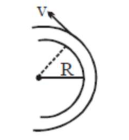# A modern grand-prix racing car of massQuestion:

A modern grand-prix racing car of mass $\mathrm{m}$ is travelling on a flat track in a circular arc of radius $\mathrm{R}$ with a speed $\mathrm{v}$. If the coefficient of static friction between the tyres and the track is $\mu_{s}$, then the magnitude of negative lift $F_{L}$ acting downwards on the car is:

(Assume forces on the four tyres are identical and $g=$ acceleration due to gravity)1. (1) $\mathrm{m}\left(\frac{\mathrm{v}^{2}}{\mu_{s} \mathrm{R}}+\mathrm{g}\right)$

2. (2) $\mathrm{m}\left(\frac{\mathrm{v}^{2}}{\mu_{s} \mathrm{R}}-\mathrm{g}\right)$

3. (3) $\mathrm{m}\left(\mathrm{g}-\frac{\mathrm{v}^{2}}{\mu_{\mathrm{n}} \mathrm{R}}\right)$

4. (4) $-\mathrm{m}\left(\mathrm{g}+\frac{\mathrm{v}^{2}}{\mu_{\mathrm{s}} \mathrm{R}}\right)$

Correct Option: , 2

Solution:

(2)

$\mu_{s} N=\frac{m v^{2}}{R}$

$N=\frac{m v^{2}}{\mu_{s} R}=m g+F_{L}$

$F_{L}=\frac{m v^{2}}{\mu_{s} R}-m g$